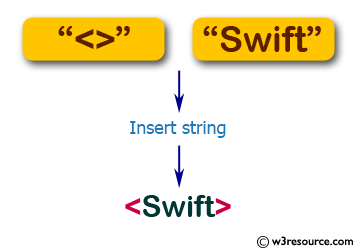﻿ Swift String Programming Exercise: Insert a given string to another given string where the second string will be in the middle of the first string - w3resource

# Swift String Exercises: Insert a given string to another given string where the second string will be in the middle of the first string

## Swift String Programming: Exercise-2 with Solution

Write a Swift program to insert a given string to another given string where the second string will be in the middle of the first string.

Pictorial Presentation:Sample Solution:

Swift Code:

``````func insert_string(_ str1: String, _ str2: String) -> String {
var current_index = str1.startIndex
let char1: Character = str1[current_index]
var result = str1

while char1 == str1[current_index] {
current_index = str1.index(after: current_index)
}

result.insert(contentsOf: str2.characters, at: current_index)

return result
}

print(insert_string("<>", "Swift"))
print(insert_string("<>>", "Swift"))
print(insert_string("[]", "Swift"))
```
```

Sample Output:

```<Swift>
<Swift>>
[Swift]
```

Swift Programming Code Editor:

Improve this sample solution and post your code through Disqus

What is the difficulty level of this exercise?

﻿

Inviting useful, relevant, well-written and unique guest posts